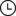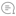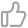如何使用GPU改善JavaScript性能IQ前端 |362021-11-28 18:49000

什么是 GPU.js

https://gpu.rocks/#/

• GPU.js 使用 JavaScript 作为基础，允许你使用 JavaScript 语法。
• 它承担着将 JavaScript 自动转译为着色器语言的责任，并对它们进行编译。
• 如果设备中没有 GPU，它可以退回到普通的 JavaScript 引擎。因此，使用 GPU.js 不会有任何不利因素。
• GPU.js 也可以用于并行计算。此外，你可以同时在 CPU 和 GPU 上异步地进行多项计算。

如何设置 GPU.js？

对于 Node 项目

npm install gpu.js --save
or
import { GPU } from ('gpu.js')
--- or ---
const { GPU } = require('gpu.js')
--- or ---
import { GPU } from 'gpu.js'; // Use this for TypeScript
const gpu = new GPU();

对于 Bowsers

<script src="dist/gpu-browser.min.js"></script>
--- or ---
<script
src="https:/
/unpkg.com/gpu.js@latest/dist/gpu- browser.min.js">
</script>
<script
rc="
https://cdn.jsdelivr.net/npm/gpu.js@latest/dist/gpu-browser.min.js">
</script>
<script>
const gpu = new GPU();
...
</
script>

创建函数

const exampleKernel = gpu.createKernel(function({
...
}, settings);

const settings = {
output: 
};

• 1D : [长度] - 值[this.thread.x]

内部支持的变量

Number

const exampleKernel = gpu.createKernel(function({
const number1 = 10;
const number2 = 0.10;
return number1 + number2;
}, settings);

Boolean

GPU.js 中也支持布尔值，与 JavaScript 类似。

const kernel = gpu.createKernel(function({
const bool = true;
if (bool) {
return 1;
}else{
return 0;
}
},settings);

Arrays

const exampleKernel = gpu.createKernel(function({
const array1 = [0.0110.110];
return array1;
}, settings);

Functions

const exampleKernel = gpu.createKernel(function({
function privateFunction({
return [0.0110.110];
}
return privateFunction();
}, settings);

支持的输入类型

Numbers

const exampleKernel = gpu.createKernel(function(x{
return x;
}, settings);
exampleKernel(25);

1D,2D, or 3D Array of Numbers

const exampleKernel = gpu.createKernel(function(x{
return x;
}, settings);
exampleKernel();

const { input } = require('gpu.js');
const value = input(flattenedArray, [width, height, depth]);

HTML Images

//Single Image
const kernel = gpu.createKernel(function(image{
...
})
.setGraphical(true)
.setOutput();

const image = document.createElement('img');
image.src = 'image1.png';
kernel(image);
document.getElementsByTagName('body').appendChild(kernel.canvas);
};
//Multiple Images
const kernel = gpu.createKernel(function(image{
this.color(pixel, pixel, pixel, pixel);
})
.setGraphical(true)
.setOutput();

const image1 = document.createElement('img');
image1.src = 'image1.png';
....
....
const totalImages = 3;
kernel([image1, image2, image3]);
document.getElementsByTagName('body').appendChild(kernel.canvas);
}
};

使用 GPU.js 的第一个功能

第 1 步，生成 1000 个元素的数组的函数

generateMatrices() {
this.matrices = [[], []];
for (let y = 0; y < this.matrixSize; y++) {
this.matrices.push([])
this.matrices.push([])
for (let x = 0; x < this.matrixSize; x++) {
const value1 = parseInt((Math.random() * 10).toString())
const value2 = parseInt((Math.random() * 10).toString())
this.matrices[y].push(value1)
this.matrices[y].push(value2)
}
}
}

第 2 步,内核函数

gpuMultiplyMatrix() {
const gpu = new GPU();
const multiplyMatrix = gpu.createKernel(function (a: number[][], b: number[][], matrixSize: number{
let sum = 0;

for (let i = 0; i < matrixSize; i++) {
}
return sum;
}).setOutput([this.matrixSize, this.matrixSize])
const startTime = performance.now();
const resultMatrix = multiplyMatrix(this.matrices,  this.matrices, this.matrixSize);

const endTime = performance.now();
this.gpuTime = (endTime - startTime) + " ms";

console.log("GPU TIME : "this.gpuTime);
this.gpuProduct = resultMatrix as number[][];
}

步骤 3,CPU 乘法函数。

cpuMutiplyMatrix() {
const startTime = performance.now();
const a = this.matrices;
const b = this.matrices;
let productRow = Array.apply(nullnew Array(this.matrixSize)).map(Number.prototype.valueOf, 0);
let product = new Array(this.matrixSize);

for (let p = 0; p < this.matrixSize; p++) {
product[p] = productRow.slice();
}

for (let i = 0; i < this.matrixSize; i++) {
for (let j = 0; j < this.matrixSize; j++) {
for (let k = 0; k < this.matrixSize; k++) {
product[i][j] += a[i][k] * b[k][j];
}
}
}
const endTime = performance.now();
this.cpuTime = (endTime — startTime) + “ ms”;
console.log(“CPU TIME : “+ this.cpuTime);
this.cpuProduct = product;
}

结论

结语

「关注公众号IQ前端，一个专注于CSS/JS开发技巧的前端公众号，更多前端小干货等着你喔」

• 关注后回复资料免费领取学习资料
• 关注后回复进群拉你进技术交流群
• 欢迎关注IQ前端，更多「CSS/JS开发技巧」只在公众号推送00

暂无评论~~
Ctrl+Enter